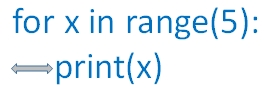# Python programming tutorials

 all() Checking elements of Iterator any() Checking elements of Iterator bin() Converting integer to Binary bool() Return True or False based on input object bytes() Returns bytes object counter() dict subclass for counting hashable objects date Date functions String String methods and applications list Ordered changeable items list tuple Ordered unchangeable items list set Unordered and unindexed list functions User defined function with Parameters handling frozenset Making elements of iterable object to immutable File handling Methods for file handling Sample Codes Sample codes in python str Converting object to string try catch Handling error in Python Class & Object Object Oriented Programming in Python iterator iterator using iter() id() Getting unique id of the object for loop for loop with break continue and else generator creating iterator in python Json Json methods to manage Json data formatting tkinter Adding GUI in Python len Number of elements present in an iterable or string map apply function to each element of iterables max Maximum value of the iterable or string or series min Manimum value of the iterable or string or series math Math functions mutable Mutable and Immutable objects reversed() Returns a reversed iterator sum Sum of all elements of an iterable zip iterator object by using different iterables random Generating random integer and float numbers between a range Django Python web framework
Operators
 in Membership Operators is Identity Operators Bitwise Bitwise operators in Python
Database Management using Python
 MySQL Python functions to manage MySQL database
Installing Python
 IIS cgi Python through IIS CGI
Pandas
 pandas Python data analysis tool

## Numpy

 NumPy Array Scientific computing with Python Array Methods Methods to get result ( output ) using NumPy random.randit Random integers with lower and upper limits and size random.rand Uniform distribution [0,1] of random numbers of given shape & population random.randn Normal distribution of random numbers of given shape & population random_sample Continuous uniform distribution over an intervalBy using BeautifulSoup we can parse HTML or XML documents and get the required tags or contents
 tensorflow tensorflow basics matplotlib matplotlib library to draw graphs BeautifulSoup matplotlib library to draw graphs

## indentation mattersBlock of codes are maintained by using same indent. Line of codes within a particular loop or if condition is maintained within the same indent. Nested loops also maintain the similar indenting condition.

## printing output with format

``````id=5
name="Ronal Jack"
mark=45
print("ID :{:02d} Name:{:15s} Mark: {:2d}".format(id,name,mark))``````
Output is here
``ID :05 Name:Ronal Jack      Mark: 45``
``````str1="Welcome "
str2=" to "
str3=" Python "
str4=" Your student id = "
id=5

print(" Hi {2} {1} {0} ".format(str1,str2,str3))
print(" Hi {0} {1} ".format(str4,id))``````
Output is here
``````Hi  Python   to  Welcome
Hi  Your student id =  5 ``````
Correct the first line to get proper sequnce of words

## Printing decimal upto 2 places using format

``````my_str="Mr ABCD"
avg=356.23456
id=5
name="Welcome to Python"

Output is here
``Hi Mr ABCD, Your id = 5, Your Average =356.23)``
Python adds one line break after each print command, we can remove the line break ( Watch the `end=''` within the `print ()`)
``````my_str="Mr ABCD"
avg=356.23456
id=5
name="Welcome to Python"

print("Hi {}, Your id = {} ".format(my_str,id),end='')
output is here
``Hi Mr ABCD, Your id = 5 Your Average =356.23)``

## Printing using variables inside string

``````a=2
b="welcome"
c="Python"
print("Hello ",b," to ", c )``````
Output is here
``Hello  welcome  to  Python``

## Using escape charLet us print I can't travel by bus
``print(' I can\'t travel by bus')``
We used escape char \ inside the word can't to print single quote instead of using as string termination.
We can use special char \ inside our string without using as escape char by adding r before the quotes
``print(r"C:\MyDocument\python\test.py")``
Here \t is not considered as Tab and the out put is here
``C:\MyDocument\python\test.py``

We can add doble quote inside single quote
``print ( 'We said "Welcome to plus2net"')``
Output is here
``We said "Welcome to plus2net"``
plus2net.complus2net.com